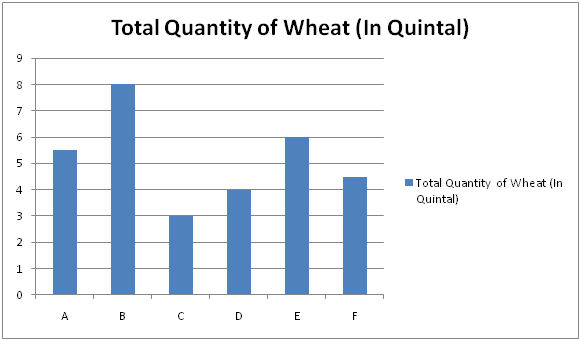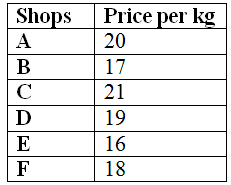# IBPS Clerk Prelims 2018 – Quantitative Aptitude Questions Day-24

Dear Readers, Bank Exam Race for the Year 2018 is already started, To enrich your preparation here we have providing new series of Practice Questions on Quantitative Aptitude – Section. Candidates those who are preparing for IBPS Clerk Prelims 2018 Exams can practice these questions daily and make your preparation effective.

[WpProQuiz 4242]

1) A, B and C started a business by investing Rs. 45000, Rs. 32000 and Rs. 28000 respectively. After 5 months, A withdraw Rs. 15000 and B invested Rs. 8000 more. And after another 3 months, C invested 25 % of initial investment more. Find the total profit at the end of the year, if the share of B is Rs. 44000?

a) Rs. 154600

b) Rs. 144200

c) Rs. 123900

d) Rs. 175800

e) None of these

2) A tank can be filled by three pipes A, B and C in 10 hours. All the pipes were opened for 2 hours and then pipe C was closed. A and B filled the remaining tank in 12 hours. Find the time taken by pipe C alone to completely fill the tank?

a) 30 hours

b) 28 hours

c) 24 hours

d) 32 hours

e) None of these

3) A boat can travel 15 km downstream in 18 min. The ratio of the speed of the boat in still water to the speed of the stream is 4 : 1. How much time will the boat take to cover 10 km upstream?

a) 24 min

b) 16 min

c) 20 min

d) 28 min

e) None of these

4) Find the difference between the compound interest on Rs. 32000 for 2 years at 5 % per annum compounded annually and compounded half yearly (approx)?

a) Rs. 49

b) Rs. 53

c) Rs. 42

d) Rs. 57

e) Rs. 61

5) The present age of A is 2 times the age of B, 6 years ago. C is 8 years elder than B and 7 years younger than A. Find the present age of B?

a) 27 years

b) 32 years

c) 25 years

d) 30 years

e) None of these

Directions (Q. 6 – 10) Study the following information carefully and answer the given questions:

The following bar graph shows the total quantity of sale of wheat (In Quintal) of 6 different shops and the table shows the price per kg of wheat.Note:

1 Quintal = 100 kg

Total amount earned = Total Quantity of Wheat sold * Price per kg

6) Total Quantity of Wheat sold by the shop A and C together is approximately what percentage of total quantity of Wheat sold by the shop B and D together?

a) 50 %

b) 85 %

c) 115 %

d) 70 %

e) 100 %

7) Find the ratio between the total amount earned by shop B to that of shop E?

a) 17 : 12

b) 23 : 28

c) 11 : 15

d) 8 : 3

e) None of these

8) Find the total amount earned by shop D, E and F together?

a) 20800

b) 22600

c) 25300

d) 27500

e) None of these

9) The total amount earned by shop B is approximately what percentage more than the total amount earned by shop C?

a) 70 %

b) 115 %

c) 160 %

d) 95 %

e) 130 %

10) Find the difference between the total quantity of wheat sold by the shop A, B and E together to that of the shop C, D and F together?

a) 10 quintal

b) 15 quintal

c) 17 quintal

d) 8 quintal

e) None of these

The share of A, B and C,

= > [45000*5 + 30000*7] : [32000*5 + 40000*7] : [28000*8 + 28000*(125/100)*4]

= > 435000 : 440000 : 364000

= > 435 : 440 : 364

440’s = 44000

1’s = 100

Total profit = 1239’s = Rs. 123900

(A + B + C)’s one hour work = (1/10)

(A + B + C)’s two hour work = (2/10) = 1/5

Remaining = 4/5

(4/5)*(A + B)’s whole work = 12

(A + B)’s whole work = 12*(5/4) = 15 hours

C’s one hour work = (1/10) – (1/15) = (15 – 10)/(15*10)

= > (1/30)

C alone can complete the tank in 30 hours

Speed of downstream = 15*(60/18) = 50 km/hr

The ratio of the speed of the boat in still water to the speed of the stream

= > 4 : 1 (4x, x)

5x = 50

X = 10

Speed of upstream = 4x – x = 3x = 30 km/hr

Distance = 10 km

Time = D/S = 10/30 = 1/3 hr = (1/3)*60 = 20 min

If the interest compounded annually,

32000*(5/100) = 1600

33600*(5/100) = 1680

C.I = 1600 + 1680 = 3280

If the interest compounded half yearly,

32000*(5/200) = 800

32800*(5/200) = 820

33620*(5/200) = 840.5

34460.5*(5/200) = 861.5125

C.I = 800 + 820 + 840.5 + 861.5125 = Rs. 3322.0125

Required difference = 3322 – 3280 = Rs. 42

The ratio of present age of A and B’s age 6 years ago = 2 : 1

The present age of A and B = 2x, x + 6

C = B + 8

C = A – 7

B + 8 = A – 7

X + 6 + 8 = 2x – 7

X + 14 = 2x – 7

X = 21

Present age of B = x + 6 = 27 years

Direction (6-10) :

Total Quantity of Wheat sold by the shop A and C together

= > 5.5 + 3 = 8.5 quintal

Total quantity of Wheat sold by the shop B and D together

= > 8 + 4 = 12 quintal

Required % = (8.5/12)*100 = 70 %

The total amount earned by shop B

= > (800*17)

The total amount earned by shop E

= > (600*16)

Required ratio = (800*17) : (600*16) = 17 : 12

The total amount earned by shop D, E and F together

= > [(400*19) + (600*16) + (450*18)]

= > [7600 + 9600 + 8100]

= > 25300

The total amount earned by shop B

= > 800*17 = 13600

The total amount earned by shop C

= > 300*21 = 6300

Required % = [(13600 – 6300)/6300]*100 = 115 %

The total quantity of wheat sold by the shop A, B and E together

= > 5.5 + 8 + 6 = 19.5 quintal

The total quantity of wheat sold by the shop C, D and F together

= > 3 + 4 + 4.5 = 11.5 quintal

Required difference = 19.5 – 11.5 = 8 quintal

Daily Practice Test Schedule | Good Luck

 Topic Daily Publishing Time Daily News Papers & Editorials 8.00 AM Current Affairs Quiz 9.00 AM Current Affairs Quiz (Hindi) 9.30 AM IBPS Clerk Prelims – Reasoning 10.00 AM IBPS Clerk Prelims – Reasoning (Hindi) 10.30 AM IBPS Clerk Prelims – Quantitative Aptitude 11.00 AM IBPS Clerk Prelims – Quantitative Aptitude (Hindi) 11.30 AM Vocabulary (Based on The Hindu) 12.00 PM IBPS PO Prelims – English Language 1.00 PM SSC Practice Questions (Reasoning/Quantitative aptitude) 2.00 PM IBPS PO/Clerk – GK Questions 3.00 PM SSC Practice Questions (English/General Knowledge) 4.00 PM Daily Current Affairs Updates 5.00 PM IBPS PO Mains – Reasoning 6.00 PM IBPS PO Mains – Quantitative Aptitude 7.00 PM IBPS PO Mains – English Language 8.00 PM